### Usage of Multivariate Distributions

The following simple example illustrates the usage of the multivariate distributions in PROC MCMC. Suppose you are interested in estimating the mean and covariance of multivariate data using this multivariate normal model: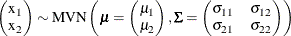where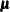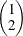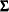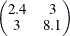You can use the following independent prior onand: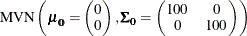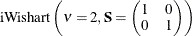The following IML procedure statements simulate 100 random multivariate normal samples:

title 'An Example that Uses Multivariate Distributions';
proc iml;
N = 100;
Mean = {1 2};
Cov =  {2.4 3, 3 8.1};
call randseed(1);
x = RANDNORMAL( N, Mean, Cov );

SampleMean = x[:,];
n = nrow(x);
y = x - repeat( SampleMean, n );
SampleCov = y*y / (n-1);
print SampleMean Mean, SampleCov Cov;

cname = {"x1", "x2"};
create inputdata from x [colname = cname];
append from x;
close inputdata;
quit;


Figure 55.13 prints the sample mean and covariance of the simulated data, in addition to the true mean and covariance matrix.

Figure 55.13: Simulated Multivariate Normal Data

 An Example that Uses Multivariate Distributions

SampleMean   Mean
0.9987751 2.115693 1 2

SampleCov   Cov
2.8252975 3.7190704 2.4 3
3.7190704 9.2916805 3 8.1

The following PROC MCMC statements estimate the posterior mean and covariance of the multivariate normal data:

proc mcmc data=inputdata seed=17 nmc=3000 diag=none;
ods select PostSummaries PostIntervals;
array data x1 x2;
array mu;
array Sigma[2,2];
array mu0 (0 0);
array Sigma0[2,2] (100 0 0 100);
array S[2,2] (1 0 0 1);
parm mu Sigma;
prior mu ~ mvn(mu0, Sigma0);
prior Sigma ~ iwish(2, S);
model data ~ mvn(mu, Sigma);
run;


To use the multivariate distribution, you must specify parameters (or random variables in the MODEL statement) in an array form. The first ARRAY statement creates an one-dimensional array data, which contains two numeric variables, x1 and x2, from the input data set. The data variable is your response variable. The subsequent statements defines two array-parameters (mu and Sigma) and three constant array-hyperparameters (mu0, Sigma0, and S). The PARMS statement declares mu and Sigma to be model parameters. The two PRIOR statements specify the multivariate normal and inverse Wishart distributions as the prior for mu and Sigma, respectively. The MODEL statement specifies the multivariate normal likelihood with data as the random variable, mu as the mean, and Sigma` as the covariance matrix.

Figure 55.14 lists the estimated posterior mean and covariance matrix.

Figure 55.14: Estimated Mean and Covariance

The MCMC Procedure

Posterior Summaries
Parameter N Mean Standard
Deviation
Percentiles
25% 50% 75%
mu1 3000 0.9941 0.1763 0.8761 0.9958 1.1136
mu2 3000 2.1135 0.3112 1.9075 2.1056 2.3254
Sigma1 3000 2.8726 0.4084 2.5799 2.8347 3.1205
Sigma2 3000 3.7573 0.6418 3.3090 3.7057 4.1385
Sigma3 3000 3.7573 0.6418 3.3090 3.7057 4.1385
Sigma4 3000 9.3987 1.3224 8.4705 9.2507 10.1946

Posterior Intervals
Parameter Alpha Equal-Tail Interval HPD Interval
mu1 0.050 0.6500 1.3356 0.6338 1.3106
mu2 0.050 1.5081 2.7405 1.4939 2.7165
Sigma1 0.050 2.1725 3.8034 2.1001 3.6723
Sigma2 0.050 2.6659 5.2064 2.5791 5.0223
Sigma3 0.050 2.6659 5.2064 2.5791 5.0223
Sigma4 0.050 7.1260 12.3763 7.0155 12.0969